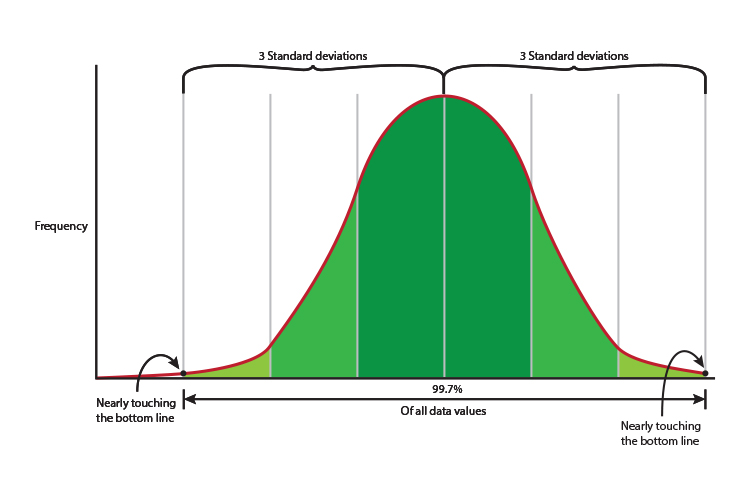# What does standard deviation really mean?

If a set of data is said to have a normal distribution then the standard deviation can be used to determine the proportion of values that lie within a particular range of the mean.

The standard deviation is usually presented in conjunction with the mean

For a normal distribution:

1. 68% of the data is less than 1 standard deviation away from the mean (1SD).
2. 95% of the data is less than two standard deviations away from the mean.
3. 99.7% of the data is less than three.

68% = 1 SD
95% = 2 SD
99.7% = 3SD

## What does 1 SD (one standard deviation) mean

On a bell curve or normal distribution of data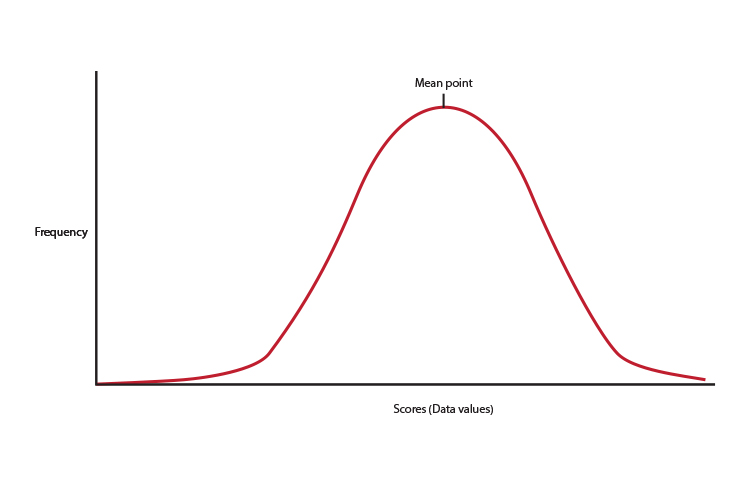1 SD = 1 Standard deviation = 68% of the scores or data values is roughly filling the area of a bell curve from a 1/3 of the way down the y axis.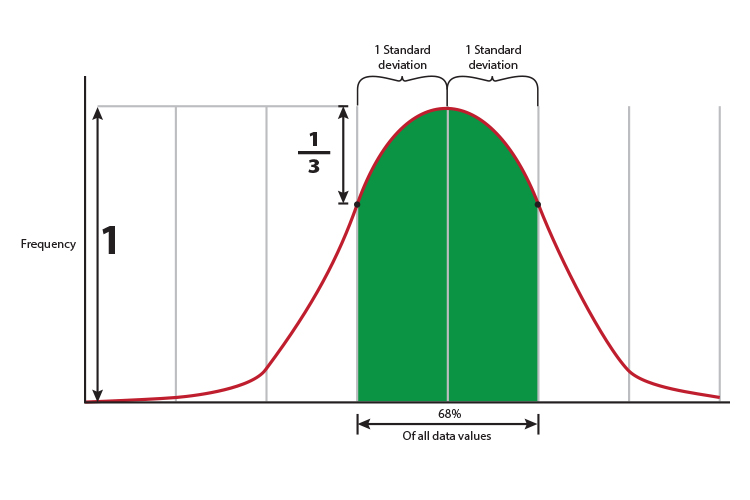## What does 2 SD (two standard deviations) mean

On a bell curve or normal distribution of data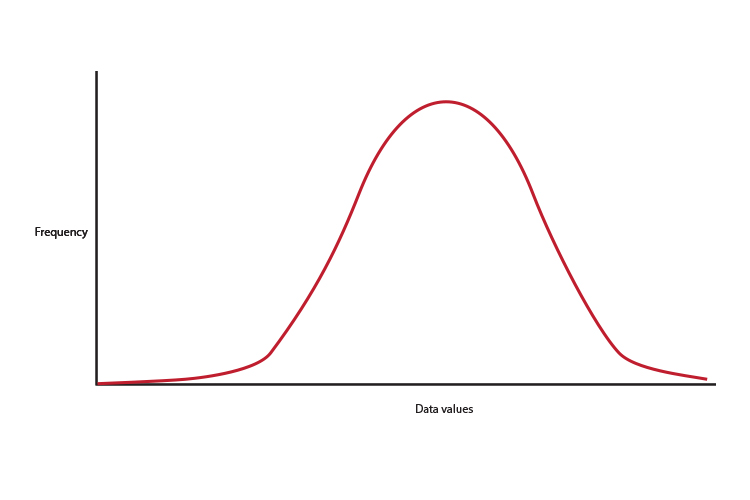2 SD = 2 Standard deviation = 95% of the scores or data values is roughly filling the area of a bell curve from nine tenths of the way down the y axis.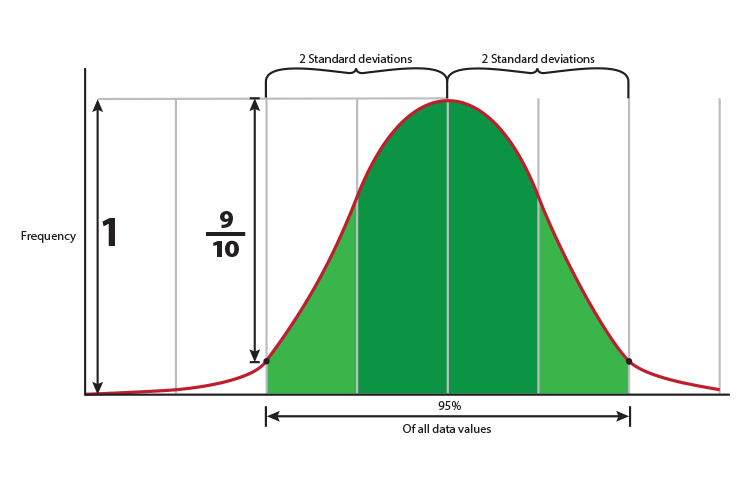## What does 3 SD (three standard deviations) mean

On a bell curve or normal distribution of data3 SD = 3 Standard deviation = 99.7% of the scores or data values is roughly filling the area of a bell curve from just before it touches the x axis.